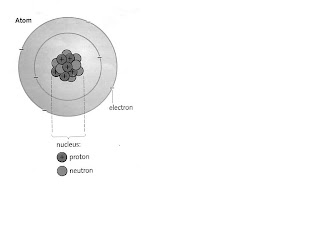## Tuesday, November 20, 2007

### ELECTRIC FIELDS AND CHARGE FLOW1. State the relationship between electron and electric current
• Matter is made up of tiny particles called atoms. At the center of the atom is the nucleus which is made up of protons and neutrons. Surrounding the nucleus are particles called electrons. A proton has a positive charge. An electron has an equal negative charge while a neutron is neutral.

A body is:

(a) neutral, if it has equal numbers of positive and negative charges.

(b) charged negative, if it has more negative than positive charges. (atom gains electron)

(c) charged positive, if it has more positive than negative charges. (atom losses electron)

· The force acting on two bodies of the same net charges will repel each other.

· The force acting on two bodies of different net charges will attract each other.

· The force causes movement of electrons or flow of charges.

Define Electric Current:

· The rate of flow of electric charge

· Current, I = charge, Q = Q

Time, t t

· The SI unit = ampere, A